## Mar 9, 2017

Source: Alok Goyal (Stellaris VP, Ex-Helion VC) puzzle blog

Problem: A fast food restaurant sells dimsums in boxes of 7 and 3. What’s the greatest number of dimsums a person cannot buy. Generalize it for p and q where p and q are relatively prime.

I loved the puzzle. Hope you enjoy it too.

1.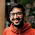3*n+1
= 3(n-2) + 6 + 1
= 3(n-2) + 7

if n >= 2, 3*n + 1 will always be comb of 3 and 7
max num of such type - 5

3*n + 2
= 3*(n-4) + 2*7

if n >= 4, 3*n + 2 will always be comb of 3 and 7
max num of such type - 11

Still thinking about generalization to p and q

2.For (7,3) combination, the answer is 11. In general it is pq - (P+Q)

3.For (7,3) combination the answer is 11. In general, it is pq-(P+Q)

4.pq-p-q is the greatest such number. Proof:

WLOG assume q > p. Say p mod q = r. First we will prove that given any x > pq-p-q, there exists a >=0, b>= 0 s.t. ap + bq = x. We prove this in two parts:

a) consider x >= pq-q = q(p-1). Say x mod p = s. Since gcd(p,q) = gcd(r,q) = 1, there exists some t with 0 <= t <= p-1 such that r*t = s mod p. Now, x >= q(p-1) >= qt, and x-qt = 0 mod p, thus, x-qt = pb for b >= 0.

b) consider x > pq-p-q but < pq-q. In this case, note that we can tighten the bound of t to be 0 <= t <= p-2, since x != r(p-1) mod p (both the limits of q(p-1)-p and q(p-1) are excluded). Thus, x > pq-p-q = q(p-1) - p >= q(t+1) - p = qt + q-p. Thus, x-qt > q-p > 0, and x-qt = 0 mod p, which implies that x-qt = pb with b > 0.

Finally, to show that pq-p-q can't be expressed as ap + bq, note that pq-p-q mod p = q(p-1) mod p. Also ap + bq = bq mod p. Thus, bq = q(p-1) mod p, which means b = p-1 mod p (since q is coprime to p). Any such b is >= p-1, but pq-p-q < q(p-1), thus it can't be expressed as ap + bq. QED.

In the given specific case, we have the minimum such number as 3*7-3-7 = 11.

5.11

3,6,9,12,15,18 ... all these can be ordered.
7,10,13,16,19... all these can be ordered.
14,17,20,23,... all these can be ordered.

So, 11 is the one which can't be ordered.

So, basic idea is to see the smallest number and try to find out all possible modular can be generated. As soon as this is achieved, we can get the largest number which can't be created.

Thanks.

6.7.8.9.Because working with mod 7:
0,3,6 are done.
For x modulo 1, we need to subtract 14 from biggest multiple of 7 less than x...i.e. x>=15 and x=1 mod 7 dimsums can be bought.
Similarly; x=2 mod 7 need to subtract 7; x=4 mod 7 need to subtract 14 and lastly x=5 mod 7 need to subtract 7.

10.i'm not sure if i understand this correctly-- but i am going to assume 'relatively prime' means that for some p, q they are consecutive prime numbers where p < q. if this is the case, i believe that the maximum number that person cannot buy using p, q is some number r = q + 1, assuming p =/=2.

if p > 2, then we know that the set of possible values for p, q are all odd numbers. Also, with (p < q), the maximum number of buys that we can buy can only be made with linear combinations of (xp, yq) where x, y are the multiplicative factor.
Aside, given that p, q are odd, and if p is not 2, p + q > q + 1; and q + 1 is even number.. So basically the even number that is larger than q cannot be represented using p, q; and the smallest number that is larger than q is q+1. I know this is not rigorous but just wanted to see if this is the right approach.

11.12.13.14.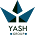15.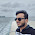This is the Frobenius Problem. The general solution for p,q is pq - p - q.

16.Lets say X is the number of dimsums which i can't buy, but I can buy (X+i) dimsums for every possible integer i.
Hence, X+1, X+2, X+3,...etc should all be of the form 7x+3y.
Now lets say X+1 is of the form 7x+3y. Then you should be able to get X+2 by either adding/removing 7s or 3s.
Now, X+3, X+6 and X+7 can easily be formed using 7s and 3s since X is of the form 7x + 3y. All I need to know is how do I add 1,2,4,5, to a number by adding/subtracting 3s and/or 7s.

So if I need a 1, I add a 7 and remove a couple of 3s. i.e. 7 - 2(3)
Similarly,
1 = 7 - 2(3)
2 = 3(3) - 7
4 = 7 - 3
5 = 7(2) - 3(3)

So if you are going from X to X+1 or X+2 or X+4 or X+5, then you need at least three 3s and at least one 7, because if you have them you can subtract them from X and add a few 7s and 3s (which are available to you anyway) and get your desired numbers.
Hence X = 3(3) + 7(1) = 16
hence the number before 16 which can't be formed using 7s and 3s should be the answer.
15= 5(3)
14 = 2(7)
13 = 7+ 2(3)
12 = 3(4)
11 = ?
Hence the answer should be 11.

17.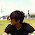Lets say X is the number of dimsums which i can't buy, but I can buy (X+i) dimsums for every possible integer i.
Hence, X+1, X+2, X+3,...etc should all be of the form 7x+3y.
Now lets say X+1 is of the form 7x+3y. Then you should be able to get X+2 by either adding/removing 7s or 3s.
Now, X+3, X+6 and X+7 can easily be formed using 7s and 3s since X is of the form 7x + 3y. All I need to know is how do I add 1,2,4,5, to a number by adding/subtracting 3s and/or 7s.

So if I need a 1, I add a 7 and remove a couple of 3s. i.e. 7 - 2(3)
Similarly,
1 = 7 - 2(3)
2 = 3(3) - 7
4 = 7 - 3
5 = 3(4) - 7

So if you are going from X to X+1 or X+2 or X+4 or X+5, then you need at least two 3s and at least one 7, because if you have them you can subtract them from X and add a few 7s and 3s (which are available to you anyway) and get your desired numbers.
Hence X = 2(3) + 7(1) = 13
hence the number before 13 which can't be formed using 7s and 3s should be the answer.

13 = 7+ 2(3)
12 = 3(4)
11 = can't be formed using 3s and/or 7s
Hence the answer should be 11.

18.19.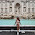11.
Every other number can be expressed as 3k, 3k+7 or 3k+14 (and so on).

20.21.22.This should be 11 for 7 and 3. The generalized answer for co-prime D and u would be x*y-(x+y)

### Fraction Brainteaser

Source: Sent to me by Gaurav Sinha Problem: Siddhant writes a Maths test and correctly answers 5 out of 6 Arithmetic questions and 20...